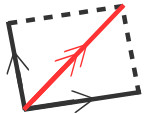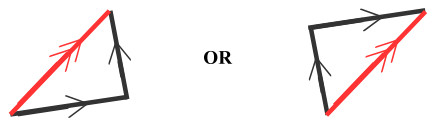Home » Handbook of O Level Physics » UNIT 3: FORCES

# UNIT 3: FORCES

• Force: It is a push or pull that one object exerts on another which produces, or tends to produce motion, stops or tends to stop motion.

• Unit: N

• Examples of forces are:

weight; tension; magnetic force; electric force; contact force; friction; resistance;

• Simply add / subtract if vectors are acting in a line.

• Law of Parallelogram:• Newton’s Laws of Motion

• First Law: Every object continues in its state of rest or uniform motion in a straight line unless a resultant force acts on it to change its state.

• Second Law: Acceleration of a mass is directly proportional to the resultant force.

F ∝ a

F = m a

Note: Acceleration has the same direction as the resultant force.

• Third Law: To every action there is an equal and opposite reaction.

• Balanced forces

• When there is no resultant force

• acceleration will be zero (2nd Law)

• no change in state of motion (1st Law)

• Unbalanced forces

• When there is a resultant force

• acceleration will NOT be zero (2nd Law)

• change in state of motion(1st Law)

• Friction: It is the force that opposes motion.

• Friction is affected by

• lubrication / oiling

• how hard the surfaces are rubbed together

• nature of the surfaces in contact

• Types

• Static friction is the friction when a body is not moving.

• Limiting friction is the (maximum static) friction when a body is just about to move.

• Dynamic friction is the friction when a body is moving.

Note: Dynamic friction is always less than limiting friction.